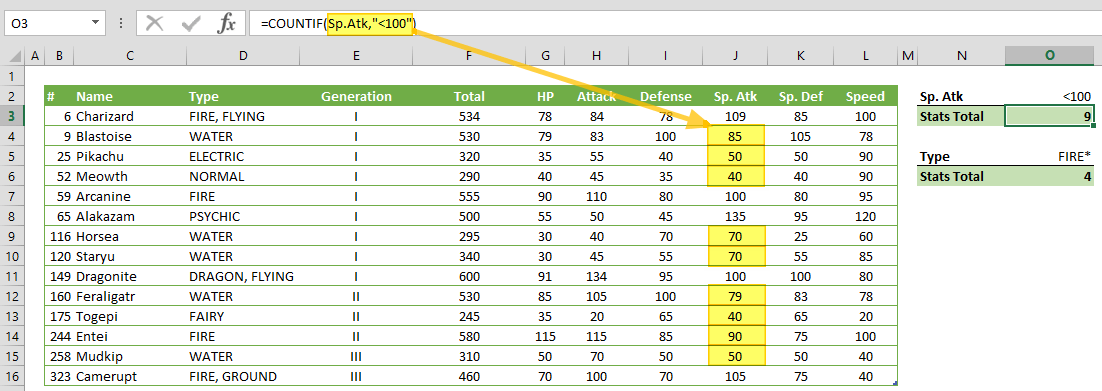COUNTIF counts the values in a range of cells that match a given criteria. In this guide, we’re going to show you how to use the COUNTIF function and also go over some tips and error handling methods.

# Supported versions

• All Excel versions

# Syntax

COUNTIF(range, criteria)

# Arguments

 range The range of cells that you want to apply the criteria against criteria The criteria to be applied to the range values, and determine which cells are to be counted.

Examples

We're using named ranges in our examples to make the formulas easier to read. You don't need to do this to run your formulas.

## Example 1

=COUNTIF(Sp.Atk,"<100")
formula counts Sp.Atk values, where Sp.Atk is less than 100.## Example 2

=COUNTIF(Type,"FIRE*",HP)
formula sums the HP values, where Type starts with the string "FIRE". If you use "FIRE" without asterisks, the COUNTIF function will skip the HP values for "FIRE, GROUND" and "FIRE, FLYING".

Note that the COUNTIF function is not case-sensitive - "FIRE" and "fire" criteria will give the same results.# Tips

Comparison operators:

 Operator Description Criteria Sample Criteria Meaning = Equal to “=10000” Equal to 10000 <> Not equal to “<>10000” Not equal to 10000 > Greater than “>10000” Greater than 10000 < Less than “>10000” Less than 10000 >= Greater than or equal to “>=10000” Greater than or equal to 10000 <= Less than or equal to “<=10000” Less than or equal to 10000 ? Takes the place of a single character “Admin?” 6-character word starts by “Admin” * Can take the place of any number of characters. “Admin*” Any number of character word starts with “Admin” ~ Use tilde in front of a  question mark or an asterisk to actually find them “Admin~*” Equal to "Admin*"

Note: Wildcards cannot be used for numeric values. Searching for a wild card character in a range of numeric values will return no matches.

# Issues

## #VALUE!

The COUNTIF function returns incorrect results when you use it to match strings longer than 255 characters, or the string #VALUE!.

## Empty Cell Reference

If criteria argument refers to an empty cell, the COUNTIF function evaluates the cell value as 0.